# Open Ended Question Types, Examples and Samples

Open-ended questions that the candidate can answer by writing, speaking, filling in tables, taking pictures, writing code, uploading files and using similar methods are essential components in many exams,tests, and assessment processes.

Open ended questions are preferred in the measurement of competencies that cannot be evaluated with multiple-choice questions or matching questions.

In open-ended questions, the candidate is asked not to choose an answer, but to create it. How the candidate will create the answer is determined by the “answering type” of that open-ended question. Open-ended questions answered by writing text or numbers can be evaluated automatically by the assessment system with the automatic evaluation rules that can be determined in advance in the system, and the answers given can also be evaluated by the tutors. If preferred, comments can be added to the answers and shared with candidates.

## Types of Open Ended Questions

Open ended question types each type of which provides different tools for candidates to construct their answer, can be categorized according to their answering methods. Candidates can compose their answers by typing, speaking, writing code, uploading files, taking photo/screenshots and filling in tables, in accordance with the type of open ended question type you choose.

### Open ended questions answered by writing text

#### Short answer type questions

Candidates are given a one-line text field where they can write their answers.

#### Essay type questions

Candidates are given a multiple-lines text field where they can write their answer.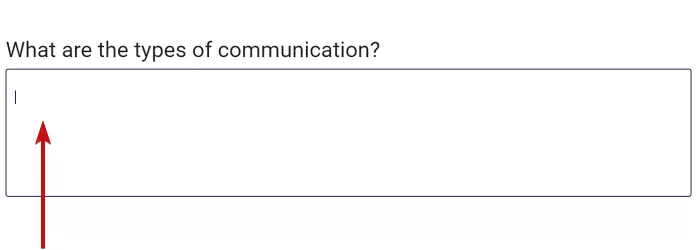Multiple-line text field offered to the candidate for questions that usually require long answers (article writing, etc.) and a sample article writing question.

#### Customizing the text field

The given text field can be made into a more easily understandable and useful format for the candidate by using many methods.

• A heading can be added to the text field.
• A temporary content can be added to the text field.
• An auxiliary content can be added to the text field.
• Short symbols or phrases can be placed at the beginning or end (prefix,suffix) of the text field.
• A character counter can be added that immediately displays the number of characters in the given answer.
• The width of the text field can be specified.
• If the text field is multi-line, the number of lines of the space to be given can be determined.
• The candidate can write from right-to-left or left-to-right.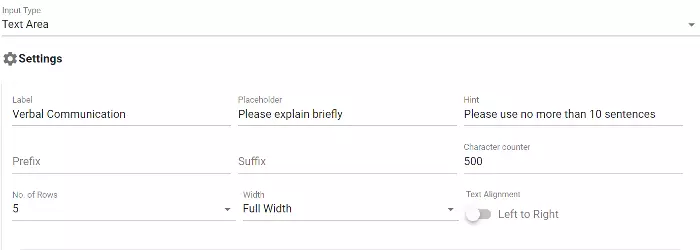Adjustment screen where the style and content of the text field to be given to candidates are customized by the admin.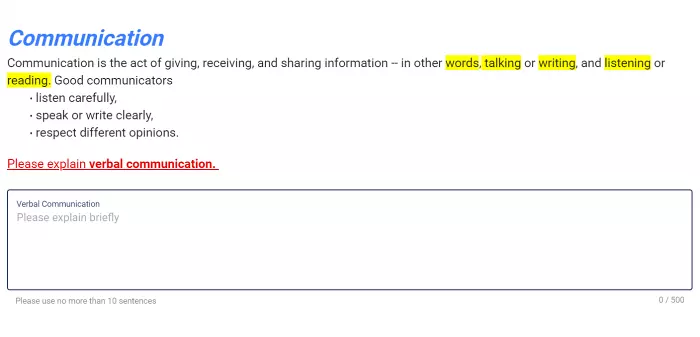Example of an open ended question customized by adding a heading, temporary content, auxiliary content, and character counter to a multi-line text field.

#### Providing a virtual keyboard enabling to answer in different languages

If you want candidates to give an answer in a certain language, but you think that they may not have a keyboard compatible with that language, a virtual keyboard can be added to the candidates' screen in the relevant language. In this way, candidates can type their answers using the keys on the virtual keyboard provided.

Virtual keyboard languages supported by the examination system:

• Turkish
• English
• Spanish
• German
• French
• Italian
• Russian
• Arabic
• Hebrew

#### Providing auxiliary keypads

If you want candidates to give an answer using the keyboard, but you want to provide a virtual keyboard in order to ensure some characters that you think may be missing on the keyboard, you can activate the auxiliary keypads.

#### Providing auxiliary numerical keypads

If you want the candidate to type numbers as the answer, you can activate a virtual keypad consisting of numbers, point or comma.

#### Limiting answers given by writing

By defining a regular expression (Regular Expression - regex), you can enforce that candidates' response conforms to a rule. If candidates write a text not complying with the rule, you can determine how you will alert them.Open-ended question example with an answer that does not match the specified regular expression and showing an automatic alert.

#### Automatic evaluation of the answer

The answer given by the candidate by writing to a question can be evaluated automatically by the system through assessing it with a rule defined by the admin.

• Equality rules:Evaluation can be made automatically, depending on whether the text written by the candidate is equal or unequal to the text defined beforehand.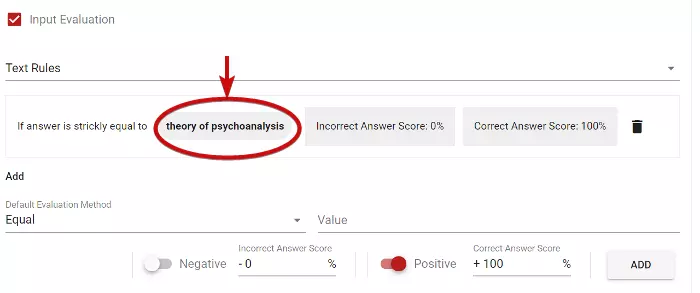Determination of the text to be used in assesing, and the grade to be given in case of equality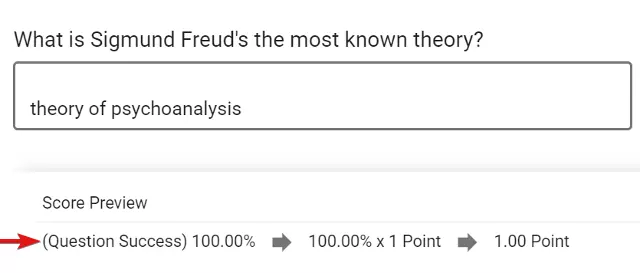A preview of an answer that matches up with the comparison text and is graded automatically.
• Equality rules for any or none: The answer given by the candidate is compared with many texts defined beforehand. If the answer is fully equal to one of texts or if it is not equal to any of them, the evaluation can be made automatically.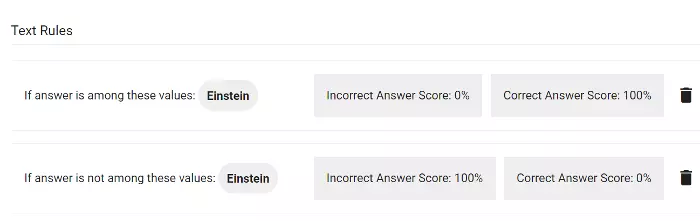Determination of the comparison texts to be used for grading automatically, and the grade to be given in case the answer is equal to any of the texts.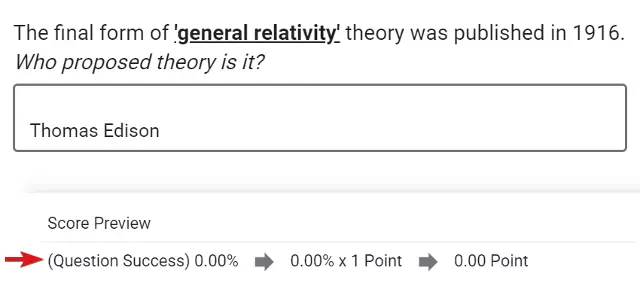Preview of the automatical scoring of an answer that is not equal to any of comparison texts
• Rules defined by regular expression: The answer given by the candidate is compared with a predefined regular expression (Regular expression-regex). Automatic evaluation can be made if it complies with the rule or not.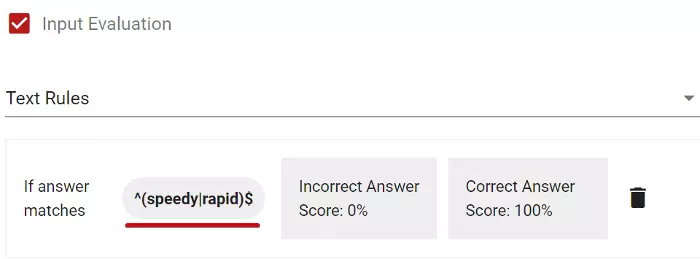Determination of the comparison rule using regex, and the grade to be given in case of a match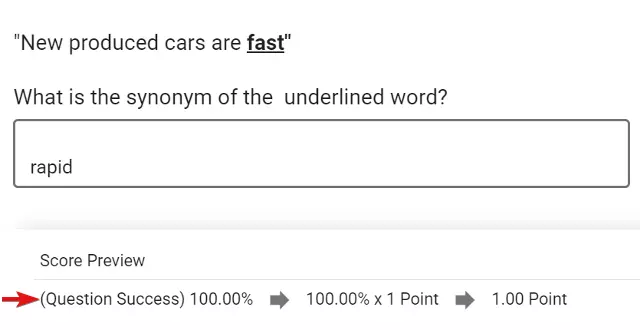A preview of an open ended question with automatic grading of the answer matching the Regex rule

#### Defining a special evaluation function to assess automatically the answer

You can define a special function that will automatically evaluate the candidate's answer. In this case, the answer given by the candidate will be automatically evaluated by the function.

### Open ended questions answered by writing numbers

If you want the question to be answered by writing a number, an open ended question answered by writing a number can be preferred. In such case, the candidate can only write one number in the answer space.

#### Customizing the number writing space

The text field of the answer can be made into a more easily understandable and useful format for the candidate by employing several methods.

• A heading can be added to the text field.
• A temporary content can be defined in the text field.
• An auxiliary content can be added to the text field.
• Short symbols or phrases can be placed at the beginning or end (prefix,suffix) of the text field.
• A character counter can be added that immediately displays the number of characters in the given answer.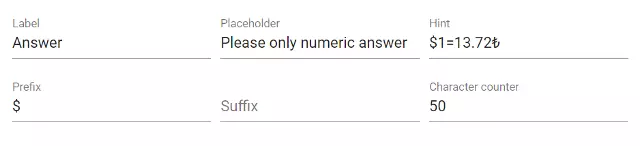Adjustment screen where the style and content of the answer field to be given to the candidate are customized by the admin.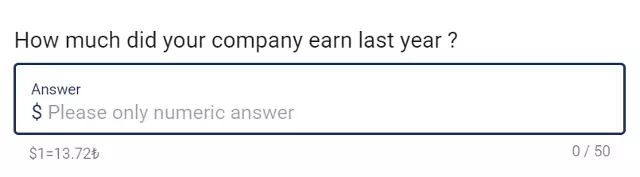An open-ended question example customized by adding a heading, prefix, temporary and auxiliary contents, and character counter to the text field.

#### Determining numerical entry method and number format

• Numerical Entry: The candidate can enter a number in accordance with your preferences for using periods and commas that you determine.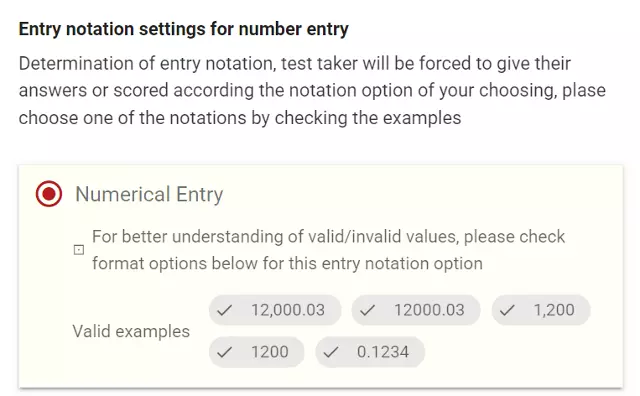Specifying the type of answer that the candidate is asked to write as Numerical Entry.An open ended question sample that is expected to be answered with a number.
• Numerical Entry with Scientific Notation:The candidate can enter a number in accordance with the rules of writing numbers in scientific notation.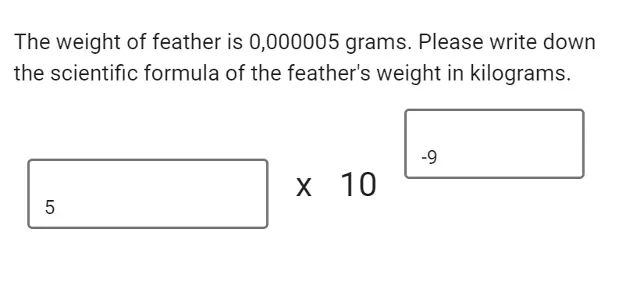An open ended question example that is expected to be answered with scientific notation.
• Numerical and Scientific Entry:The candidate can enter an answer using either numerical or scientific writing.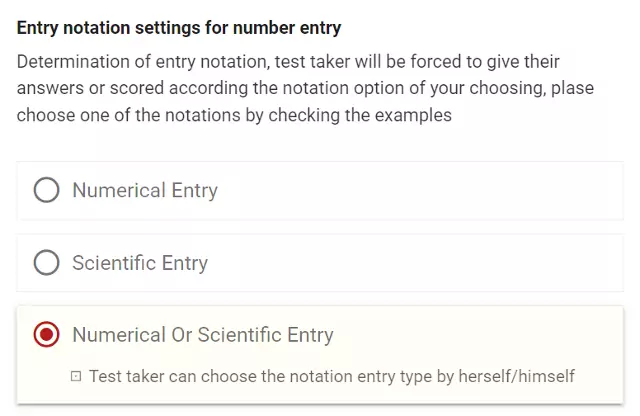Option to confirm both types of notation for the candidate's requested answerAn open ended question sample that candidates can choose the notation they want to use to answer the question.

#### Specifying thousand and precision separators to use while entering numbers

When the candidate writes a number to answer the question, it can be determined which punctuation marks to be used as thousand and precision separator.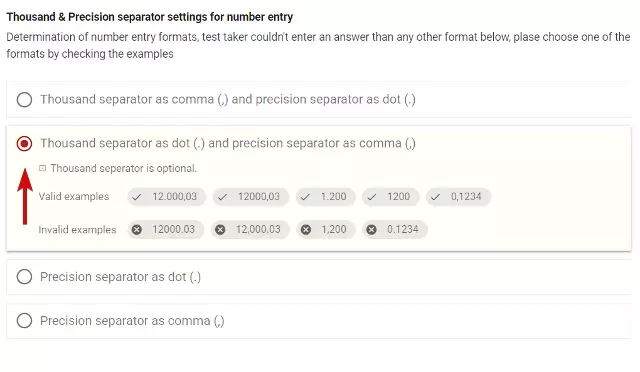Thousand and precision separator selections screenAn open ended question that includes a reminder for thousand and precision separators

#### Specifying the maximum precision digit for the numerical entries

The maximum precision of the numbers can be specified by the admin. In this way, the number that the candidate will write can consist of as many digits after the comma (or after the point) as admin determines.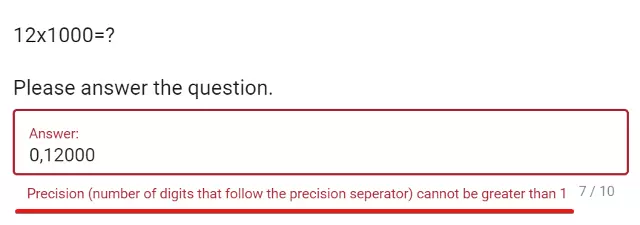Open ended question sample which gives an error message when the answer does not meet the maximum precision determined by admin

#### Limitation of the interval of numbers that can be written as answer

By defining a regular expression (Regular Expression - regex), you can enforce that candidates2 answers conform to a rule. If a text that does not comply with the rule is written, you can determine how to alert candidates.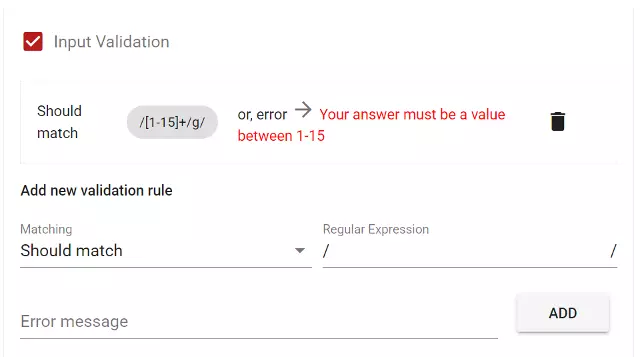Setting up as a rule with Regex the answer format requested in the question and adding an alert message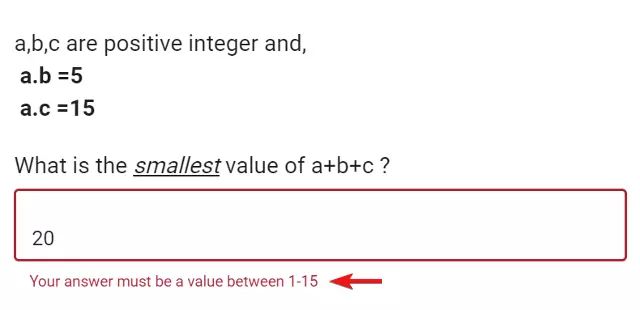Open ended question sample where an alert message given as the answer of the candidate does not comply with the rule

#### Automatic evaluation of the answer given as a number

The answer can be evaluated automatically through comnparing it with a rule defined by the admin.

• Equality rules: Evaluation can be made automatically, depending on whether the answer written by the candidate is equal or not equal to the number defined beforehand.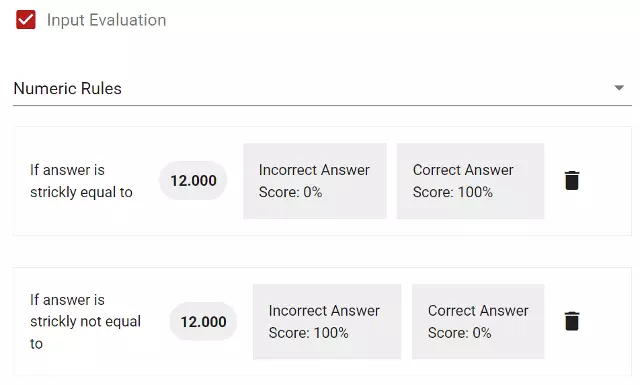Defining equality rule and grading method for the evaluation of the answer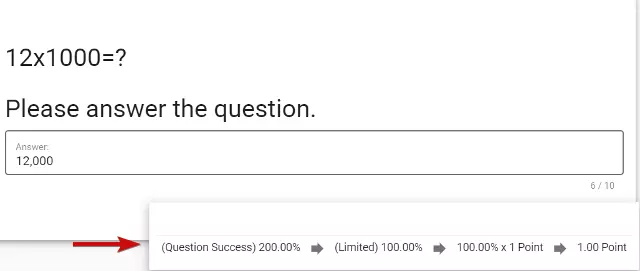An answer that conforms to the rule and is evaluated automatically.
• Equality rules for any or none: The answer given by candidates is compared with different numbers defined beforehand by the admin. The evaluation can be made automatically, dependengin on whether answer fully matches with one of the ruels or not.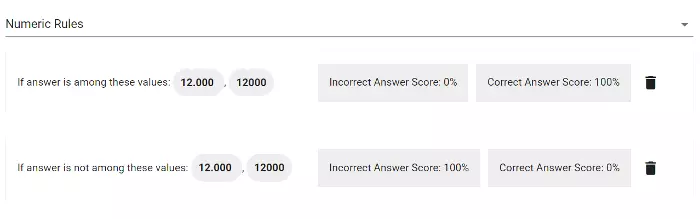Defining several values with which answer should match and the grading method according to the match rate
• Rule of being in a number interval: Evaluation can be made automatically by determining whether the number written by the candidate is within a number interval defined by the admin.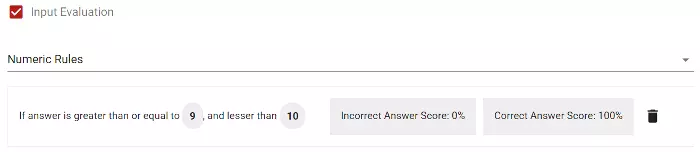Determination of the number interval where the answer given by the candidate should be and the grading method when this condition is met.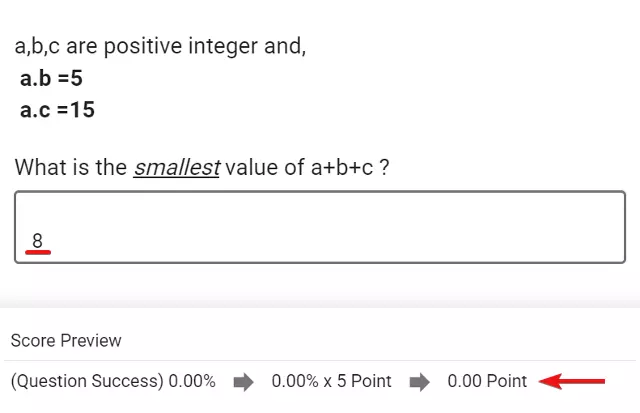Determination of the grading when the answer is not within the interval
• Rules defined by regular expression: The number written by the candidate is compared with a predefined regular expression (Regular expression - regex). An automatic evaluation can be made depending on whether the answer complies with the rule or not.

#### Defining a custom evaluation function to automatically evaluate typed number

You can write a function that will evaluate automatically the candidate's answer. In this case, the answer given by the candidate will be evaluated automatically by the function.

### Open ended questions answered by filling a table

You can create a table, specify the number of rows and columns in the table, and open spaces that the candidate can answer by typing in the desired cells of the table. You can define a single-line text space or a multi-line text space for each cell in the table.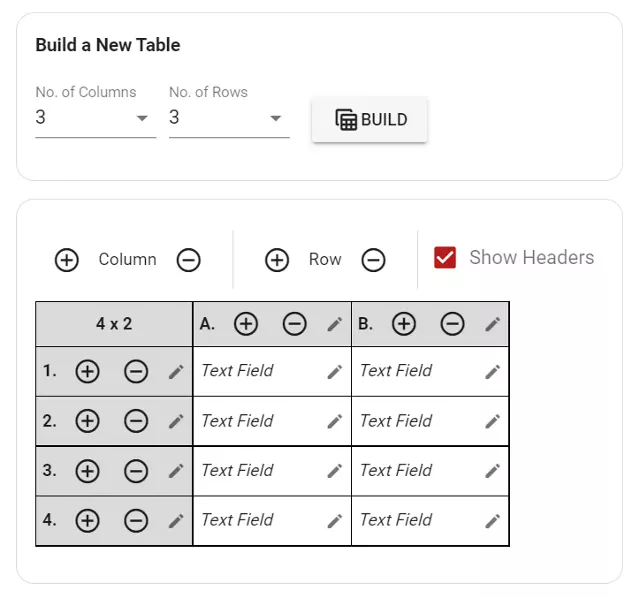Screen for editing the number of rows and columns of the table while creating a question with a table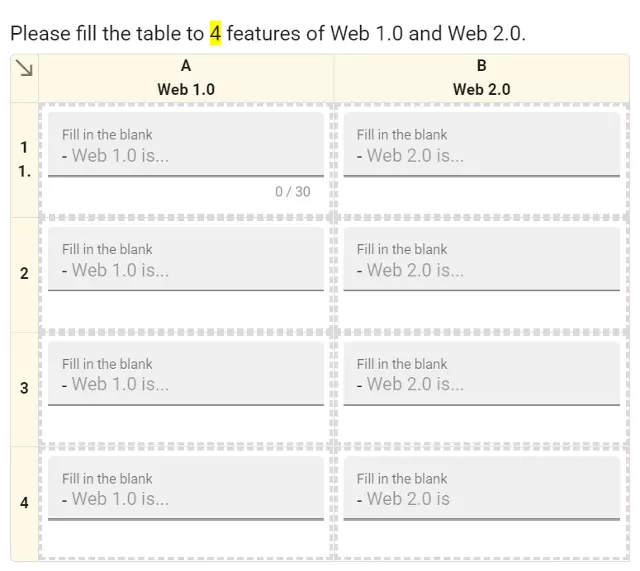An open ended question sample that the candidate is expected to answer it by filling in a table

#### Adding fixed contents to cells in a table

You can add fixed contents to cells in the table, in order to render it more understandable.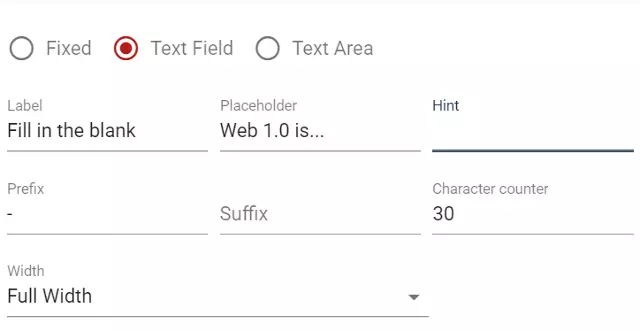Adding fixed content to cells in the table to provide information to the candidate.

#### Displaying Column and Row Headings

You can add a heading to each row and column in the table. In this way, you can make your table more clear.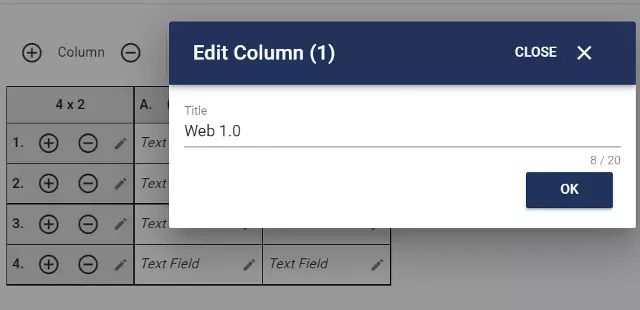Adding a heading to one of the columns in the table to be shown to the candidate

### Open ended questions answered by creating content

Candidates gives an answer to the question by creating content. They use the rich content editor to create content.

Following permissions can be granted in the answer creation process:

• Adding image: Candidate adds an image file to the content they prepared.
• Adding audio: Candidate adds an audio file to the content they prepared.
• Adding video: Candidate adds a video file to the content they prepared.
• Adding file: Candidate adds a file to the content they prepared.

You can provide the candidate with a virtual keyboard in the question creation process.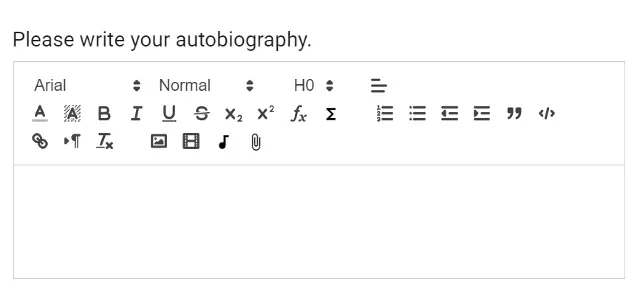An open ended question sample that the candidate is asked to answer the question by creating a content

### Open ended questions answered by recording audio (speaking questions)

Candidates answer the question by recording their voice. For this, they are provided with a voice recording application allowing them to record their voice while speaking.

• A time limit can be determined for the recording
• The number of how many times the candidate can do recording can be limited by the admin.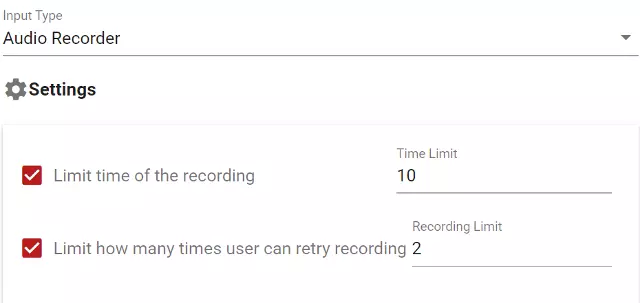The screen showing the maximum duration of the audio recording that candidates will take and how many times they have right to try recording.

### Open ended questions answered by recording a video (interview questions)

Candidates answer the question by recording their voice and image together via the webcam on their device. For this, they are provided with a video recording application where they can record by their camera.

• A time limit can be determined for the recording
• The number of how many times the candidate can do recording can be limited by the admin.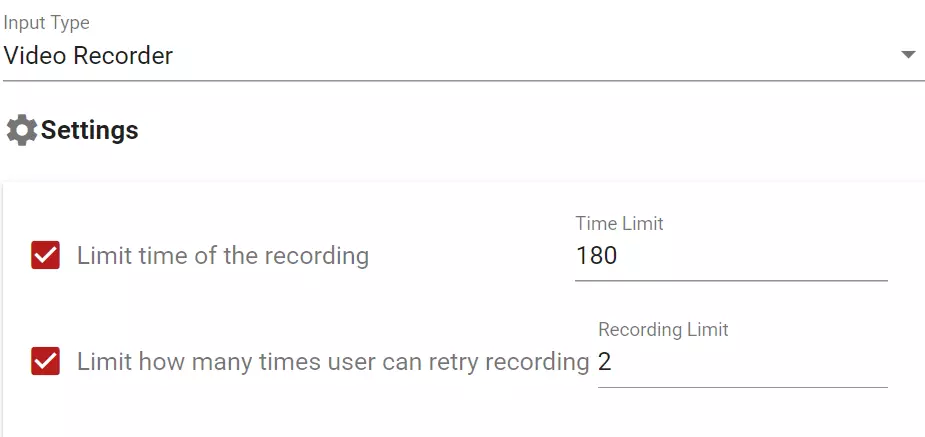The screen showing the maximum duration of the video recording that candidates will take and how many times they have right to try recording.An open ended question example that candidates answer it by recording a video with the webcam of their device.

### Open ended questions answered by writing code

Candidates answer the question by writing code in a programming language determined by admin. A code editor compatible with the chosen programming language is provided so that candidates can write their answers.

In the code editor which is provided, you can show an initial content. For this, you can save the question by adding the code you want in the editor.

#### Determining the programming language or script of the code editor

You can choose among more than 50 language options such as TypeScript, JavaScript, C#, Java, SQL, Python, Ruby, PHP, C, C++ for the code editor.

You can make candidates answer the question by uploading a file. Candidates upload the file they prepared as an answer by using the file upload tool.

### Open ended questions answered by taking a photo or uploading a screenshot

You can make candidates answer the question by uploading a screenshot on their computer, or by taking a photo via a camera connected to their devices.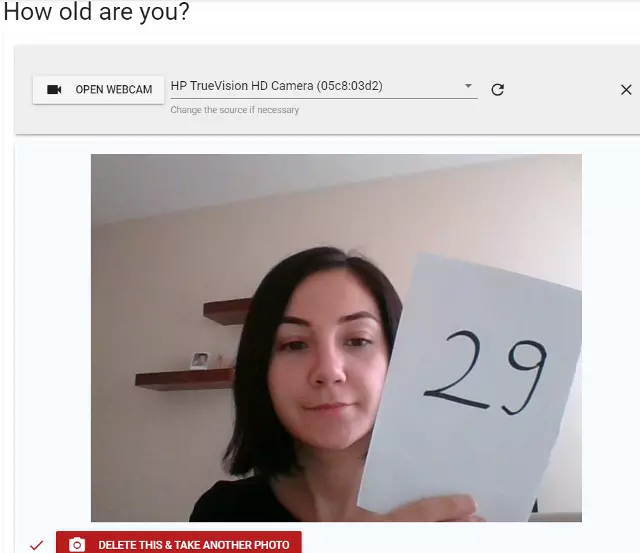An open ended question sample that candidates answer by taking a photo with the camera of device they use

## Evaluation of the Answers by the Tutors

Answers given to open-ended questions can be evaluated by tutors after the exams are completed. When an exam consisting of open-ended questions is completed, the system indicates how many questions need to be evaluated in the relevant exam.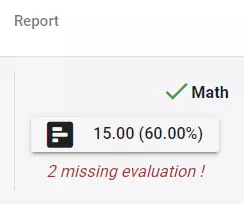Each exam in the results list has the number of questions pending to be evaluated.

After the answers are evaluated, the exam report is automatically updated.

There are 2 methods for evaluating the answers of open-ended questions. These methods can be determined from the test settings either to effect all questions, or one by one for each question in the result reports.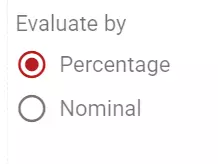The evaluation method of open ended questions either by the nominal or by the percentage can be selected from the settings for that test.
• The answer can be evaluated out of 100. In this case, after the evaluation, the exam results are updated by multiplication of the score of the question and the evaluation rate of the answer given to the question. For instance, if the score of the question in the exam is 5 and the answer given to the question is evaluated as 40% correct (successful), the candidate will receive 2 points (5 x 40%) from this question.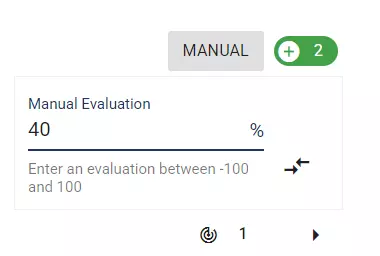Grading a question with percentage in the result reports
• A fixed grade can be determined for the answer. For instance, if the grade determined for the question is five, then the answer given by the candidate can be graded starting from a maximum of 5.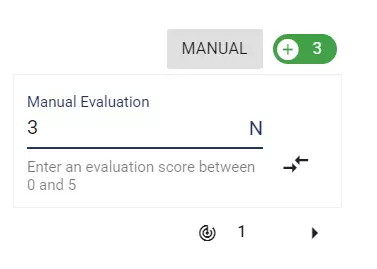Grading a question with nominal points in the result reports

### Giving feedback for the answers

A commentary content can also be included in the evaluation report of the open-ended questions. The comment may include an explanation of the evaluation, as well as listing the correct and incorrect factors in the answer, clarifying which parts of the answer are incorrect and why they are, and how the correct answer should be.